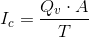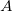# Chemical Injection Rate (Volume)

Calculates the chemical injection rate by measuring the quantity of chemical to be applied by volume. Learn more about the units used on this page. For more information go here for a fact sheet on calculating chemigation injection rates for volume-based application targets (example: pints/acre).

Desired Chemical Application:
Area to be applied to:
Injection Time:
Chemical Injection Rate:

## The Equation

When calculating the injection rate by volume use this equation.Where:= Chemical injection rate in gallons per hour (gph)= Quantity of the chemical to apply by volume. measured in gallons per acre (gal/arce)= Area measured in acres (acres)

WSU Prosser - IAREC, 24106 N Bunn Rd, Prosser WA 99350-8694, 509-786-2226,Contact Us